• 无人车路径规划算法matlab+python代码.rar
• 上一篇博客 "无人车路径规划算法—（1）算法体系综述 " 中介绍了无人车路径规划通常采用的"前端生成路径->后端平滑优化"的pipeline，本文将为大家介绍路径规划算法常用地图格式，文章主要分为以下三个模块： ...
上一篇博客 "无人车路径规划算法—（1）算法体系综述 " 中介绍了无人车路径规划通常采用的"前端生成路径->后端平滑优化"的pipeline，本文将为大家介绍路径规划算法常用地图格式，文章主要分为以下三个模块：

常用地图格式
栅格地图
拓扑地图与高精度地图

生成一个随机二维栅格地图方法的代码将开源在博主github主页

地图（MAP）
无人车的规划模块是联通感知模块，定位模块与控制模块的中间模块（架构图见图一），因此，基于搜索的规划算法通常是在能够包含无人车自身定位信息以及周边环境信息的数据结构体中进行运算，这种能够体现无人车周边环境信息的数据载体统称为地图（MAP），在介绍搜索具体的搜索算法之前，需要先引入搜索地图的概念以及常用的地图类型。本部分将重点说明以下四种常见形式：

Point Cloud Map（点云地图）
Metric Map(度量地图)
Topological Map(拓扑地图)
Semantic Map(语义地图)

1. Point Cloud Map（点云地图）
说到自动驾驶的地图，大家常最先想起的就是激光雷达给出的点云地图，但是原始点云地图通常不会被拿来进行路径规划使用，主要是由于以下原因：

点云地图通常规模很大，需要大量的存储空间，同时点云地图提供了很多不必要的细节
处理多个点云重叠处的效果不好

因此，需要利用costmap或者octomap进一步的处理（见第二部分）。
2. Metric Map（度量地图）
度量地图框架是最常见的框架，它考虑的是目标对象的二维空间。用精确的坐标表示目标对象以及各个目标之间的相对关系。这种表示方法非常有效，但其对噪声敏感，并且很难计算距离精度。譬如在地图中，北京可以用固定的经纬度来表示，上海可以用另一个固定的经纬度来表示，两者之间的距离可以通过各自的经纬度推算出来。
通常我们用稀疏（Sparse）与稠密（Dense）对它们进行分类：1

稀疏地图：对地图信息进行一定程度的抽象，只保留需要关注的信息，对次要信息进行简化
稠密地图：对所有地图中所有见到的信息进行描述，对于无人车的路径规划模块来说，稠密地图是十分必要的，通常我们将所有的有效信息通过设定的分辨率，分割到每个固定的栅格中（二维的栅格称为Grid,三维的栅格称为Voxel）。每个栅格由占有，空闲，与未知三种状态构成以表述对应栅格的障碍物占据情况。但是我们也看到，这种地图需要存储每一个格点的状态，耗费大量的存储空间，而且多数情况下地图的许多细节部分是无用的。

在度量地图中，与无人车规划模块或机器人导航模块应用最广泛的则是占据栅格地图（Occupancy Grid Map）与代价地图（CostMap）
2.1 Occupancy Grid Map (占据栅格地图)
占据栅格地图是把环境划分成一系列栅格，其中每一栅格给定一个可能值，表示该栅格被占据的概率。占据栅格的构建主要是基于二值贝叶斯滤波原理利用多传感器（激光雷达，相机等）的输入判断地图内每个栅格的障碍物占据概率。
由于占据栅格地图通常是单层结构，更适用于简单的路径规划功能，当机器人或无人车对于环境信息精确度要求不高时可以利用此地图进行规划，当规划模块对精度与安全性要求较高时，更好的选择是利用代价地图（CostMap）进行规划。
2.2 CostMap (代价地图)
官方的说明文档中有以下两幅图需要重点关注：

地图结构说明图 （见图一）
障碍物膨胀（inflation）与栅格分数关系图（见图二）

下图（图一）可以大致描述出costmap地图中的三个主要组成要素：

Footprint （红色多边形）
障碍物的膨胀层 （蓝色栅格）
障碍物位置 （红色栅格）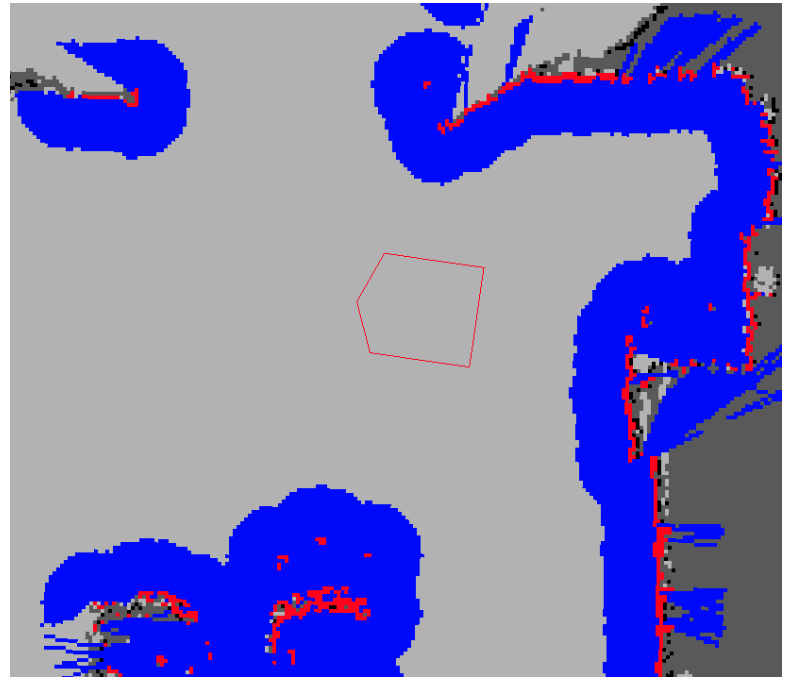下图（图二）详细的解释了障碍物膨胀区栅格分数的取值依据，其中红色多边形为车辆轮廓：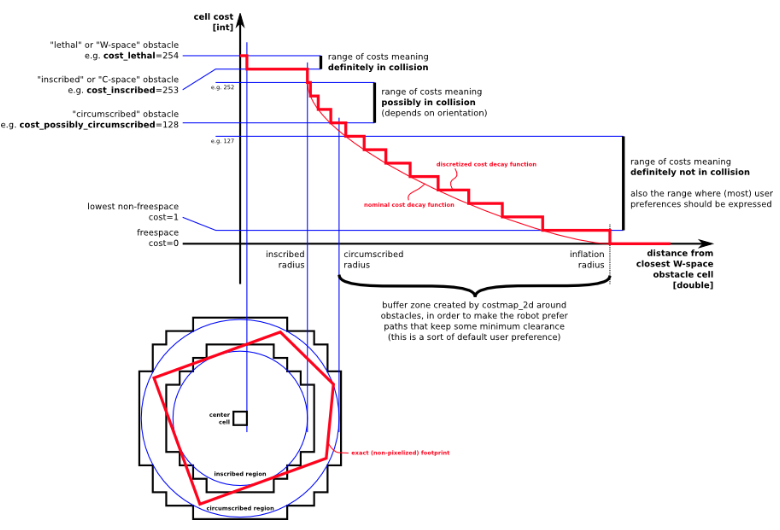Lethal：机器人的中心与障碍物所占据栅格重合，此时机器人必然与障碍物冲突
Inscribed：障碍物所占据栅格与机器人footprint内切圆重合，此时机器人必然与障碍物冲突
Possibly circumscribed：机器人外接圆与障碍物占据栅格相切或接近，因此是否碰撞取决于机器人的朝向等因素
Freespace：自由空间，无碰撞风险
Unknow：未知区域
其他的栅格的分数可以根据与Lethal以及Freespace的距离来计算，也可以根据自身产品设计相应的衰减函数来推算

介绍完costmap自身构建原理之后，另外一个值得注意的重点则是栅格地图坐标系与world坐标系转换问题：

单层地图由许多个cell组成，一般可以认为单层地图有两个坐标系：位置坐标系(以m为单位)和索引坐标系(以cell为单位)。在地图构造完成后，会有索引坐标系到位置坐标系的转换关系，所以在定位某个cell的时候可以使用任意坐标系，不存在本质性的区别。

转换关系如下图所示2：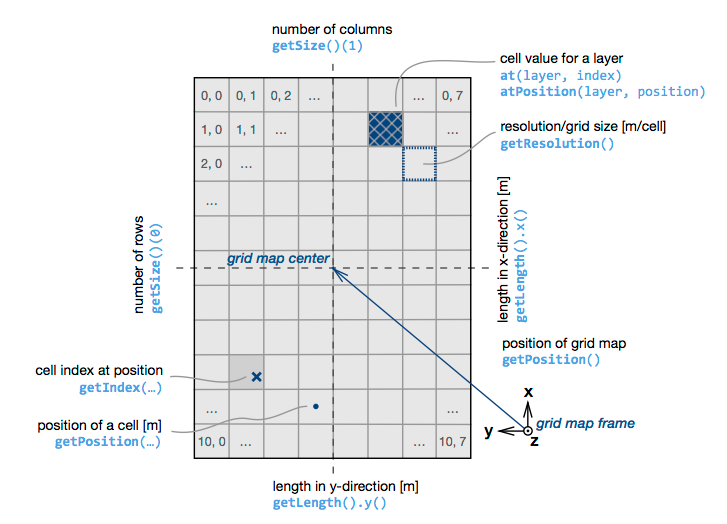地图坐标系（map frame，坐标系原点即map center，其在world中的位置就是mapPosition，X正向上，Y正向左)
内存坐标系（原点为内存块的左上角，即第一个存储的cell，可以近似理解为图像坐标系，X正向下，Y正向右）

样例代码片段如下：
/**
*@ This Section Is For Transform pose from world frame to index frame
*@ This Is The First Method
*/
gemoetometry_msgs::Pose transformPose(const geometry_msgs::Pose& pose, const tf::Transform& tf)
{
// convert ROS pose to TF pose
tf::Pose tf_pose;
tf::poseMsgToTF(pose, tf_pose);

// transform pose
tf_pose = tf * tf_pose;

// convert TF pose to ROS pose
geometry_msgs::Pose ros_pose;
tf::poseTFToMsg(tf_pose, ros_pose);

return ros_pose;
}

void poseToIndex(const geometry_msgs::Pose& pose, int* index_x, int* index_y)
{
tf::Transform orig_tf;
tf::poseMsgToTF(costmap_info.origin, orig_tf);
geometry_msgs::Pose pose_new = transformPose(pose, orig_tf.inverse());

*index_x = pose_new.position.x / costmap_info.resolution;
*index_y = pose_new.position.y / costmap_info.resolution;
}

/**
*@ This Section Is For Transform pose from world frame to index frame
*@ This Is The Second Method
*/
void poseToIndex(const geometry_msgs::Pose& pose, int* index_x, int* index_y)
{
*index_x = (pose.position.x - costmap_.info.origin.position.x) / costmap_.info.resolution;
*index_y = (pose.position.y - costmap_.info.origin.position.y) / costmap_.info.resolution;
}

/**
*@ This Section Is For Transform pose from index frame to world frame
*/
void indexToPose(int index_x , int index_y , geometry_msgs::Pose& pose)
{
double px, py;
px = costmap_.info.origin.x + (*index_x + 0.5) * costmap_.info.resolution;
py = costmap_.info.origin.y + (*index_y + 0.5) * costmap_.info.resolution;
pose.position.x = px;
pose.position.y = py;
}

2.3 开源库分享
grid_map 开源库：

grid_map库是设计有ROS接口的C++库，用于管理具有多个数据层的二维栅格地图。它是为移动机器人地图设计的，用于存储(海拔)高度、方差、颜色、摩擦系数、可通过性等数据。它用于为粗糙地形导航设计的以机器人为中心的高程地图包中。
链接：https://github.com/ANYbotics/grid_map

Octomap 开源库：

Octomap是SLAM领域经常用到的地图结构，它以八叉树数据结构来存储三维环境的概率占据地图，采用此结构可以节省大量的存储空间同时可以实现地图的压缩与更新，并且地图的分辨率可调。
链接：https://github.com/OctoMap/octomap

2.4 式例代码
编写了一个随机生成障碍物的二维地图 (跳票中。。。。最近活太多。。。正在努力填坑中，后面也会找时间把这段时间做的东西整理一下分享出来)
3 Topological Map （拓扑地图）
拓扑地图则更强调地图元素之间的关系。拓扑地图是一个图（Graph），由节点和边组成，只考虑节点间的连通性。它放松了地图对精确位置的需要，去掉地图的细节问题，是一种更为紧凑的表达方式。这种地图更适用于低纬度的路径规划，因此接触的不多，有经验的网友欢迎补充。
4 Semantic Map （语义地图）
高精度地图主要服务与自动驾驶车辆（用于机器人太奢侈，高精度地图成本太高），通过一套独特的导航体系，帮助自动驾驶解决系统性能问题，扩展传感器检测边界。它可以帮助车辆找到合适的行车空间，还可以帮助Planner确定不同的路线选择。如APOLLO中，高精度地图可以帮助车辆识别准确的道路中心线，这样在规划时就可以让车辆尽可能沿道路中心线行驶。同时在十字路口等交叉路口也可以帮助车辆缩小规划范围，以便节省计算成本与时间，在最有范围内选择出最佳路线。
参考文献：
1《视觉SLAM十四讲：从理论到实践》
2 https://github.com/FleverX/grid_map


展开全文• DOI: 10. 3969 / j. issn. 1009-9492. 2019. 05. 003朱泽凡，曾碧. 基于多线激光雷达的无人车...广东省重大科技专项项目(编号：2016B010108004)基于多线激光雷达的无人车路径规划算法*朱泽凡，曾碧(广东工业大学计算...


DOI: 10. 3969 / j. issn. 1009-9492. 2019. 05. 003朱泽凡，曾碧. 基于多线激光雷达的无人车路径规划算法［J］ . 机电工程技术，2019，48(05)：11-14.* 国家青年科学基金项目(编号61701122)；广东省重大科技专项项目(编号：2016B010108004)基于多线激光雷达的无人车路径规划算法*朱泽凡，曾碧(广东工业大学计算机学院， 广东广州510006)摘要：针对在非结构路面条件下无人车路径规划存在建模难度大，计算量较大，算法运行的实时性较差等问题，提出一种基于多线激光雷达的无人车路径规划算法。对原始点云数据进行预处理，减少需要处理的点云数量，降低程序运行复杂度；将三维点云降维形成较低分辨率的二维栅格地图，降低路径规划程序的计算量；基于构建的局部栅格地图，使用改进的人工势场法完成路径规划。算法已在无人车上应用，实验证明了该方法的有效性和可行性。关键词：非结构路面；激光雷达；三维点云；无人车；路径规划；势场法中图分类号：TP242 文献标识码：A 文章编号：1009－9492 ( 2019 ) 05－0011－04Unmanned Vehicle Path Planning Algorithm Based on Multi-Line LidarZHU Ze-fan，ZENG Bi(School of Computer，Guangdong University of Technology，Guangzhou 510006，China)Abstract: Aiming at the problems of difficult modeling， large amount of calculation and poor practicality of the algorithm in path planning of unmanned vehicle on unstructured pavement，an unmanned vehicle path planning algorithm based on multi-line lidar was proposed. The original point cloud data were preprocessed to reduce the number of point clouds needed to be processed and the complexity of the program. The three-dimensional point cloud was reduced to form a two-dimensional raster map with lower resolution， which reduced the computational complexity of the path planning program. Based on the local raster map，the improved artificial potential field method was used to complete the path planning. The algorithm has been applied in unmanned vehicle，and the experiment proves the validity and feasibility of the method.Key words: unstructured pavement；laser radar；3D point cloud；unmanned vehicle；path planning；potential field method引言      无人车路径规划技术，是指基于采集的传感器数据，完成对无人车运行环境的建模，基于构建的环境模型，完成路径规划，依据路径规划结果，控制无人车到达目标位置。      目前，在无人车路径规划研究中，常用的算法有A*算法、遗传算法、蚁群算法、粒子群优化算法、人工势场算法、神经网络算法、强化学习算法[8-9]。其中，人工势场法技术原理简单，具有计算量小，需要的数据信息少等特点，在机器人实时避障和路径规划中被广泛应用。      使用遗传算法对势场法进行改进，对运行轨迹进行优化，解决了部分场景下运行轨迹出现弯折角度过大的问题，缩短了起始点到达目标点的路径长度。混合势场法引入可视图法，在势场法无法计算出理想的运行轨迹的区域，使用可视图法确定局部目标点，控制机器人到达局部目标点后，使用势场法继续完成运行轨迹的计算。      基于模糊逻辑的改进人工势场法，在避障算法无法脱离障碍物区域时，在势场力之外，基于模糊逻辑对运行轨迹添加一个新的约束。针对障碍物处在机器人和目标点之间这一场景，对势场产生的斥力的方向进行更改，从而避免这一情况下运行轨迹无法到达目标位置。      但由于无人车运行在非结构路面，非结构路面的建模复杂度较高，计算量较大，不能将势场法直接应用于非结构路面下无人车的路径规划。      本文针对非结构路面建模难度大、计算量较大、算法运行的实时性不能保证的问题，将三维点云降维形成二维的包含障碍物的栅格地图，基于栅格地图，将改进的人工势场法应用于无人车的路径规划中，最后通过实验验证了算法的有效性和可行性。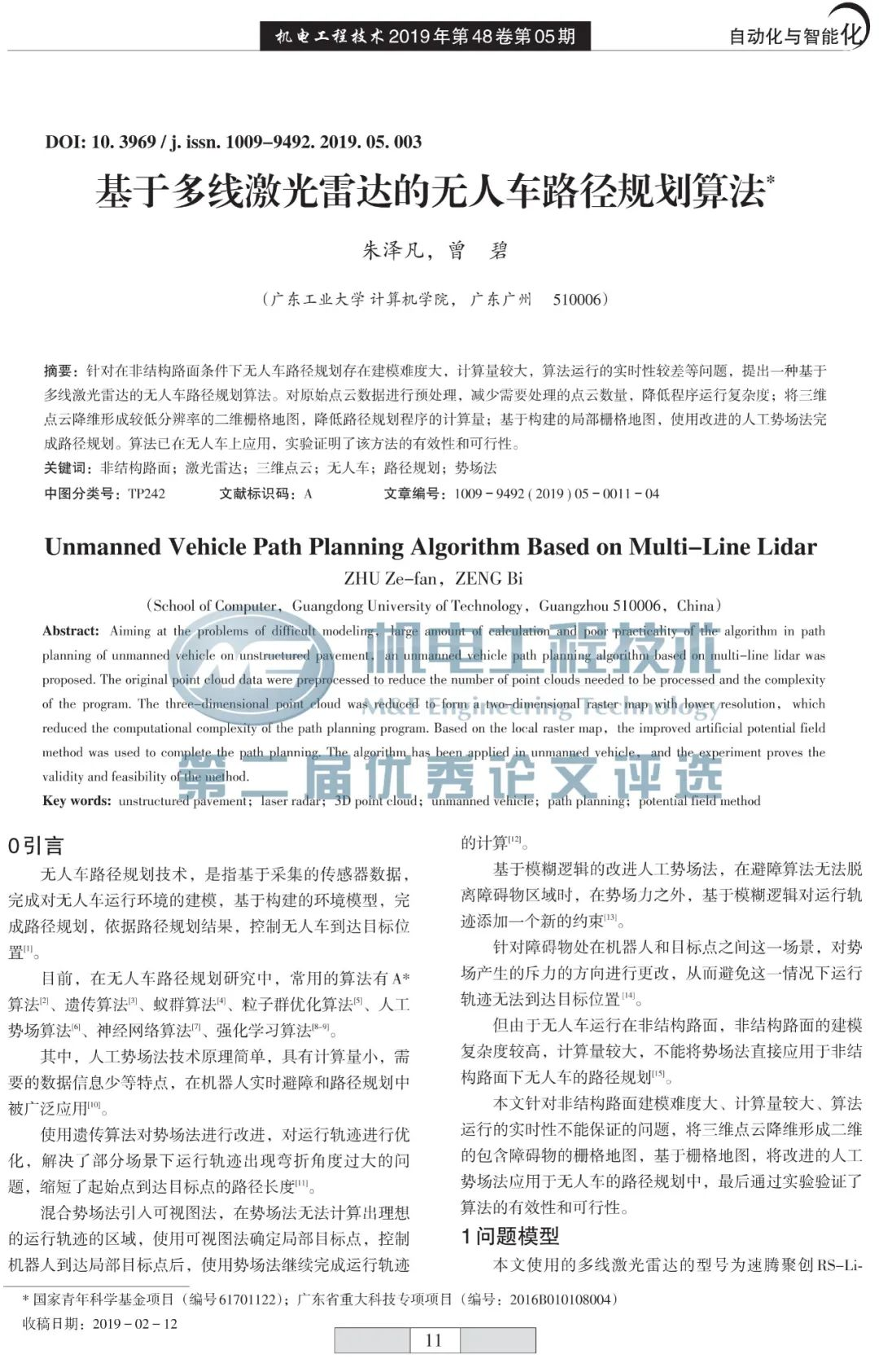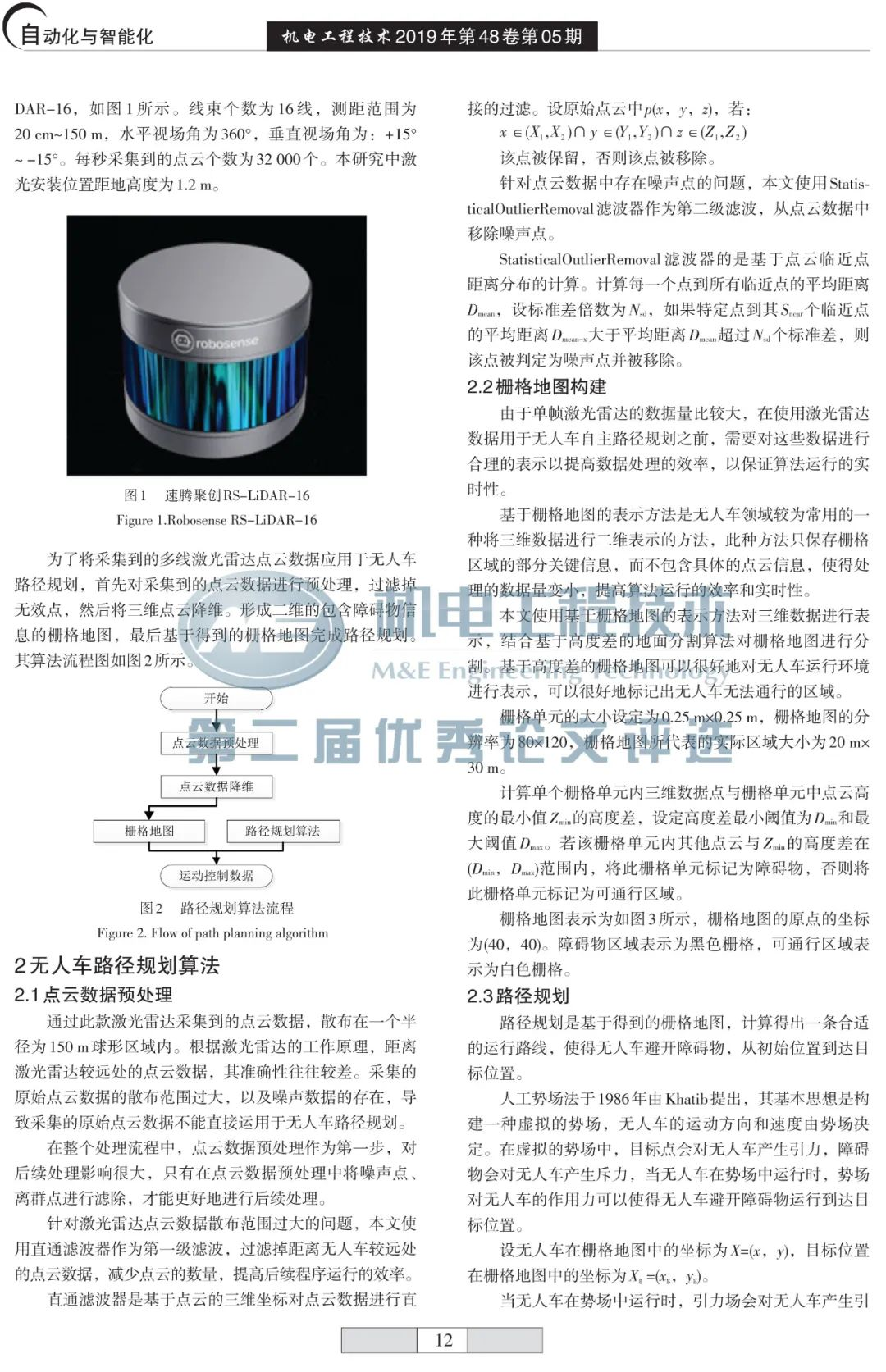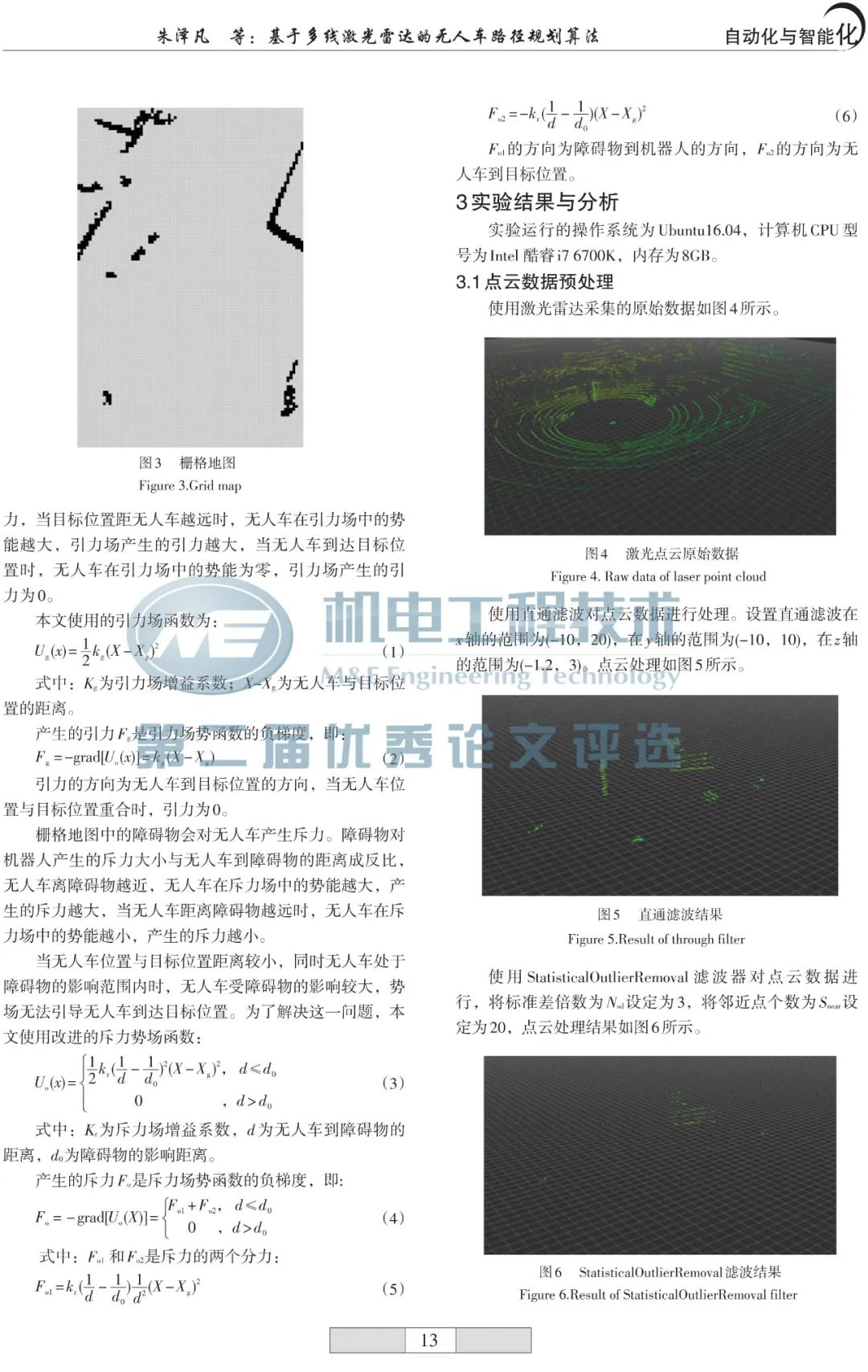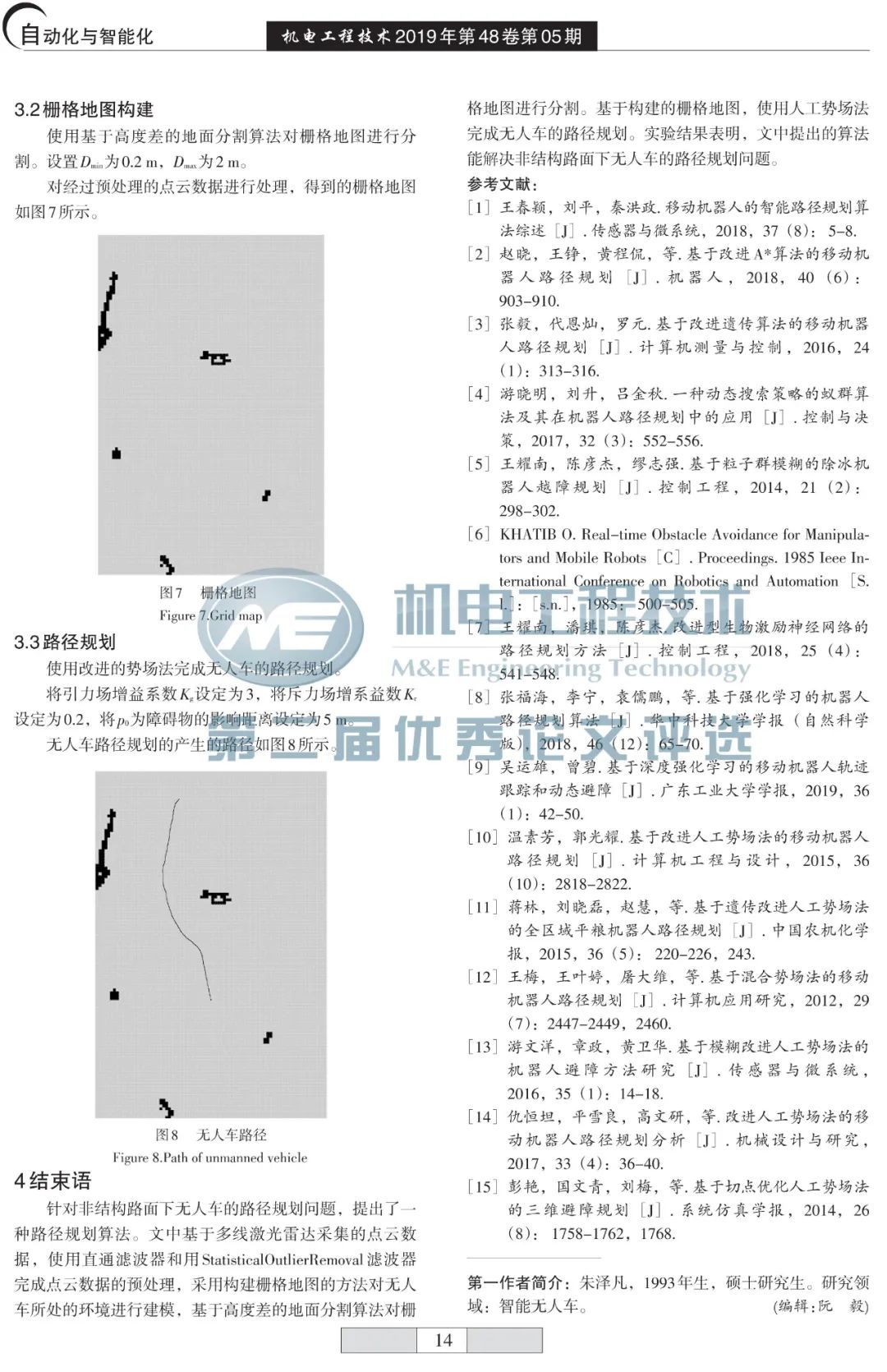结束语      针对非结构路面下无人车的路径规划问题，提出了一种路径规划算法。文中基于多线激光雷达采集的点云数据，使用直通滤波器和用StatisticalOutlierRemoval滤波器完成点云数据的预处理，采用构建栅格地图的方法对无人车所处的环境进行建模，基于高度差的地面分割算法对栅格地图进行分割。基于构建的栅格地图，使用人工势场法完成无人车的路径规划。实验结果表明，文中提出的算法能解决非结构路面下无人车的路径规划问题。欢迎投稿！欢迎转载！欢迎订阅！欢迎刊登广告！欢迎项目合作！赶快来分享关注吖↓论文在线投稿系统↓展开全文• ***写此系列博客的目的，是希望将无人车路径规划算法成体系的总结展述出来，同时分享一些常用的算法库，源代码，以及与规划层联系紧密的感知层，定位层，控制层的基础知识，可能编写跨越周期较长，自身水平有限，若...
版权声明：本文为博主原创文章，原创不易, 转载请联系博主。
写此系列博客的目的，是希望将无人车路径规划算法成体系的总结展述出来，同时分享一些常用的算法库，源代码，以及与规划层联系紧密的感知层，定位层，控制层的基础知识，可能编写跨越周期较长，自身水平有限，若有不到之处，希望得到各位的批评指正。
通用规划方法（Old-School Pipeline）是指：

首先在前端利用图搜索等方法搜索出一条初始无碰撞的安全路径（低维，离散空间）
再在后端对已生成路径进行优化以获得一条可执行的优化轨迹（高维，连续空间）
Mission -> Path Finding -> Trajectory Optimization -> Plan…

Path Finding 模块(Front-End)
主要通过构建地图进行搜索的方法或者基于采样的方法来实现前端搜索的目的：

在栅格图中（典型样例如costmap）进行图搜索，最早的是利用BFS(深度优先搜索方法),DFS（深度优先搜索方法）在建立好的图或者树中进行搜索，后来发展为经典的图搜索方法譬如利用Dijkstra,A*,D*，JPS等方法在栅格中进行搜索
利用PRM方法进行图构建（Learning Phase）,再利用Dijkstra或者A*方法进行搜索（Query Phase）
利用RRT及其衍生的算法（RRT或者informed RRT）进行边建树采样边搜索的方法

但是此类的方法存在的问题是：

如果Path Finding模块仅仅搜索简单的路径信息，把所有的轨迹优化的工作都放至路径优化模块的话，会极大的增加路径优化模块的工作量以及工作难度（Coarse to Fine原则）
轨迹往往只能在局部进行优化，若初始搜索路径的方向与当前车辆的运动方向相反，则会增加车辆的轨迹优化难度
规划出的路径若不考虑车辆运动学等约束，则常常对非完整系统没有意义，譬如无人车是无法侧向平移的，若不考虑运动学约束，常常会规划出需要侧向平移的轨迹

因此为了搜索出更加合理的前端规划路径，业界提出了结合车辆运动学约束的前端轨迹优化方法，其中典型的如State Lattice Search算法  , 斯坦福2010年提出的Hybrid Astar算法 
:Optimal Rough Terrain Trajectory Generation for Wheeled Mobile Robots, Thomas M. Howard Alonzo Kelly
:Practical Search Techniques in Path Planning for Autonomous Driving , Dmitri Dolgov, Sebastian Thrun

Trajectory Optimization模块（Back-End）
通常由前端规划方法得到的路径在平滑性，安全性等要素上仍不能满足无人车行驶的要求，因此需要对生成的路径进行进一步的优化。
常用的优化方法主要是基于最优化原理或者说凸优化方法的相关优化算法：

minimum jerk
minimum snap
此类算法常常结合自身约束条件，周围环境约束条件，目标函数等将问题转换为二次规划的问题进行求解

下一章将介绍路径规划常用地图


展开全文• A*算法搜索路径 %%寻路 Matrix(Spoint(1),Spoint(2))=0; Matrix(Epoint(1),Epoint(2))=inf; G=Matrix; F=Matrix; openlist=Matrix; closelist=Matrix; parentx=Matrix; parenty=Matrix; openlist(Spoint(1),Spoint...
%% Title: A_Star_4_Path% Authors: ruogu7% Email: 380545156@qq.com% Start time: 8:30 am,Dec 11th,2019% Latest update: 12th,Dec,2019%% Calculation steps% 1. Read .xls file and visualize points data% 2. Path routing% 3. Smoothing the path% 4. Segment the smoothed path into minimal steps, and calculate the slope% rate of each step.% 5. Visualize the result generated by steps above% 6. Make a vedio or GIF file to record the dynamic process%% 1. 初始化参数close all;clc;clear;% 地图大小m = 30;n = 30;% 起始位置Spoint = [3 3];  %起始点坐标Epoint = [29 22];%目标点坐标%% 2. 构建地图% -inf表示不可到达的障碍物点%%构建地图for i = 1:m+2if i == 1for j = 1:n+2Matrix(i,j) = -inf;endelseif i == m+2for j = 1:n+2Matrix(i,j) = -inf;endelsefor j = 1:n+2if ((j == 1)|(j == n+2))Matrix(i,j) = -inf;elseMatrix(i,j) = inf;endendendend%%障碍for j=2:10Matrix(5,j)=-inf;for j=2:15Matrix(24,j)=-inf;for j=9:24%for j=6:24Matrix(10,j)=-inf;for j=20:31Matrix(15,j)=-inf;for j=5:20Matrix(20,j)=-inf;for j=18:27Matrix(28,j)=-inf;for i=2:6Matrix(i,18)=-inf;for i=17:20Matrix(i,5)=-inf;for i=23:25Matrix(i,20)=-inf;for i=13:17Matrix(i,13)=-inf;endendendendendendendendendend% 显示地图%subplot(2,2,1);h1 = plot(Spoint(1),Spoint(2),'gO');hold on;h2 = plot(Epoint(1),Epoint(2),'rO');title('Route planing with A* algorithms');% axes('pos',[.1 .6 .5 .3])% [bottomleftcornerXposition bottomleftcornerYposition width height]% imshow('coins.jpg');annotation('arrow', [.3 .5], [.6 .5]);%% 3. A*算法搜索路径%%寻路Matrix(Spoint(1),Spoint(2))=0;Matrix(Epoint(1),Epoint(2))=inf;G=Matrix;F=Matrix;openlist=Matrix;closelist=Matrix;parentx=Matrix;parenty=Matrix;openlist(Spoint(1),Spoint(2)) =0;%closelist(Epoint(1),Epoint(2))=inf;for i = 1:n+2for j = 1:m+2k = Matrix(i,j);if(k == -inf)%subplot(2,2,1);h3 = plot(i,j,'k.');%         elseif(k == inf)  % show green feasible point%             %subplot(2,2,1);%             plot(i,j,'gh');%         else%             %subplot(2,2,1);%             plot(i,j,'gh');endhold onendendaxis([0 m+3 0 n+3]);%subplot(2,2,1);plot(Epoint(1),Epoint(2),'b+');%subplot(2,2,1);plot(Spoint(1),Spoint(2),'b+');while(1)num=inf;for p=1:m+2for q=1:n+2if(openlist(p,q)==0&&closelist(p,q)~=1)Outpoint=[p,q];if(F(p,q)>=0&&num>F(p,q))num=F(p,q);Nextpoint=[p,q];endendendendcloselist(Nextpoint(1),Nextpoint(2))=1;for i = 1:3for j = 1:3k = G(Nextpoint(1)-2+i,Nextpoint(2)-2+j);if(i==2&&j==2|closelist(Nextpoint(1)-2+i,Nextpoint(2)-2+j)==1)continue;elseif (k == -inf)G(Nextpoint(1)-2+i,Nextpoint(2)-2+j) = G(Nextpoint(1)-2+i,Nextpoint(2)-2+j);closelist(Nextpoint(1)-2+i,Nextpoint(2)-2+j)=1;elseif (k == inf)distance=((i-2)^2+(j-2)^2)^0.5;G(Nextpoint(1)-2+i,Nextpoint(2)-2+j)=G(Nextpoint(1),Nextpoint(2))+distance;openlist(Nextpoint(1)-2+i,Nextpoint(2)-2+j)=0;% H=((Nextpoint(1)-2+i-Epoint(1))^2+(Nextpoint(2)-2+j-Epoint(2))^2)^0.5;%欧几里德距离启发函数H_diagonal=min(abs(Nextpoint(1)-2+i-Epoint(1)),abs(Nextpoint(2)-2+j-Epoint(2)));%比较复杂的对角线启发函数H_straight=abs(Nextpoint(1)-2+i-Epoint(1))+abs(Nextpoint(2)-2+j-Epoint(2));H=sqrt(2)*H_diagonal+(H_straight-2*H_diagonal);% H=max(abs(Nextpoint(1)-2+i-Epoint(1)),abs(Nextpoint(2)-2+j-Epoint(2)));%比较简单的对角线函数F(Nextpoint(1)-2+i,Nextpoint(2)-2+j)=G(Nextpoint(1)-2+i,Nextpoint(2)-2+j)+H;parentx(Nextpoint(1)-2+i,Nextpoint(2)-2+j)=Nextpoint(1);parenty(Nextpoint(1)-2+i,Nextpoint(2)-2+j)=Nextpoint(2);else distance=((i-2)^2+(j-2)^2)^0.5;if(k>(distance+G(Nextpoint(1),Nextpoint(2))))k=distance+G(Nextpoint(1),Nextpoint(2));% H=((Nextpoint(1)-2+i-Epoint(1))^2+(Nextpoint(2)-2+j-Epoint(2))^2)^0.5;  %欧几里德距离启发函数H_diagonal=min(abs(Nextpoint(1)-2+i-Epoint(1)),abs(Nextpoint(2)-2+j-Epoint(2)));%比较复杂的对角线启发函数H_straight=abs(Nextpoint(1)-2+i-Epoint(1))+abs(Nextpoint(2)-2+j-Epoint(2));H=sqrt(2)*10*H_diagonal+10*(H_straight-2*H_diagonal);% H=max(abs(Nextpoint(1)-2+i-Epoint(1)),abs(Nextpoint(2)-2+j-Epoint(2)));%比较简单的对角线函数F(Nextpoint(1)-2+i,Nextpoint(2)-2+j)=k+H;parentx(Nextpoint(1)-2+i,Nextpoint(2)-2+j)=Nextpoint(1);parenty(Nextpoint(1)-2+i,Nextpoint(2)-2+j)=Nextpoint(2);endendif(((Nextpoint(1)-2+i)==Epoint(1)&&(Nextpoint(2)-2+j)==Epoint(2))|num==inf)parentx(Epoint(1),Epoint(2))=Nextpoint(1);parenty(Epoint(1),Epoint(2))=Nextpoint(2);break;endendif(((Nextpoint(1)-2+i)==Epoint(1)&&(Nextpoint(2)-2+j)==Epoint(2))|num==inf)parentx(Epoint(1),Epoint(2))=Nextpoint(1);parenty(Epoint(1),Epoint(2))=Nextpoint(2);break;endendif(((Nextpoint(1)-2+i)==Epoint(1)&&(Nextpoint(2)-2+j)==Epoint(2))|num==inf)parentx(Epoint(1),Epoint(2))=Nextpoint(1);parenty(Epoint(1),Epoint(2))=Nextpoint(2);break;endendP=[];s=1;while(1)if(num==inf)break;end%subplot(2,2,1);h4 = plot(Epoint(1),Epoint(2),'b+');P(s,:)=Epoint;s=s+1;% pause(1);xx=Epoint(1);Epoint(1)=parentx(Epoint(1),Epoint(2));Epoint(2)=parenty(xx,Epoint(2));if(parentx(Epoint(1),Epoint(2))==Spoint(1)&&parenty(Epoint(1),Epoint(2))==Spoint(2))%subplot(2,2,1);plot(Epoint(1),Epoint(2),'b+');P(s,:)=Epoint;break;endendP(s+1,:)=Spoint;legend([h1,h2,h3,h4],'Start Point','End Point','Obstacle','Routing');count=0;for i=2:12for j=2:12if(G(i,j)~=inf&&G(i,j)~=-inf)count=count+1;endendend% count%% 4. 路径优化%将得到的折现曲线拟合成光滑的曲线P=P';a=[];b=[];a=P(1,:);figure%subplot(2,2,3);plot(a,b);axis([0,n+3,0,n+3]);title('Route planing');values = spcrv([[a(1) a a(end)];[b(1) b b(end)]],3);%% 5. 结果可视化figure%subplot(2,2,4);plot(values(1,:),values(2,:),'r');axis([0,m+3,0,m+3]);title('Route planing after smoothing');
展开全文• 本文介绍了应用于无人驾驶汽车路径规划中全局路径规划的A*算法,从规划结果出发,分析传统A*的缺陷,并提出16邻域改进算法。为提高规划效率,减少路径规划时间提出双向16邻域改进算法。并与24邻域及48邻域算法进行比较,...
• 无人驾驶汽车路径规划概述 原地址：http://imgtec.eetrend.com/blog/2019/100018447.html 无人驾驶汽车路径规划是指在一定的环境模型基础上，给定无人驾驶汽车起始点和目标点后，按照性能指标规划出一条无碰撞、能...
• 用C++语言编写无人车倒车入库的路径规划，谁有这方面的资料可供查阅参考吗？真心谢谢了
• 基于目标快速探索随机树的无人驾驶汽车路径规划算法研究论文
• 单体智能的无人驾驶系统，根据功能可划分为不同的子模块，包括：高精度地图、定位模块、感知模块、预测模块、全局路径规划模块、运动规划模块、运动控制模块以及人机交互模块等。本文研究的主要内容是无人驾驶汽车...人工智能
• 文章针对近年来的无人驾驶汽车路径规划算法进行总结和归纳。首先对目前主流的环境建模方法进行阐述;其次对路径规划算法进行介绍,通过分析其优缺点,指出融合轨迹规划算法具有最好的适用性;最后总结当前研究挑战并提出...
• 在各类产业智能化发展的21世纪中，无人驾驶技术作为IT产业与传统汽车紧密相结合的代表，得到了高速发展，无人驾驶的路径规划问题一直是其发展过程中的一个难关。
• 无人驾驶路径规划论文It was a year ago (summer 2019) when I was deciding what topic to choose for my bachelor thesis. At the time I was finishing an internship at the European Space Agency where I ...算法
• 无人驾驶车辆局部路径规划的时间一致性与鲁棒性研究研究论文
• 蚁群优化算法是解决无人驾驶汽车路径规划问题的有效途径。 首先，建立无人车道规划的环境模型，处理和描述环境信息，最后实现问题空间的划分。 接下来，描述蚁群算法的仿生行为。 蚁群算法已通过添加惩罚策略进行了...
• 无人驾驶汽车在未来的智能交通系统中有着无限的想象，因此，不仅各大汽车...单体智能的无人驾驶系统，根据功能可划分为不同的子模块，包括：高精度地图、定位模块、感知模块、预测模块、全局路径规划模块、运动规划...
• 有很多同学在我们微信群和我们阿木实验室论坛提问，无人机/无人车路径规划和避障应该怎么入手。随着无人系统的发展，无人系统的路径规划的研究也越来热。生活中无人机器系统也越来越多的出现，从无人机，到无人车...
• 来源| 知乎知圈 |进“高精度地图社群”，请加微信15221054164，备注地图导读：本文选自知乎，作者详细介绍了基于深度强化学习(Deep Reinforcement Learning, DRL)的无人车自适应路径规划方法，并在无障碍环境、静态...td宽度自适应
• 运用多点预瞄与滚动优化相结合的模型预测控制算法设计了汽车的跟随...在双移线工况下进行了多组速度的跟随实验，结果表明该控制器跟随路径的误差小，对速度的适应性强。与Carsim控制器的跟随结果相比，其跟随效果更好。
• 本文提出了一种路径规划方法，该方法可在先验信息最少的情况下在室内危险区域内导航无人地面车辆。 该算法可以推广到任何给定的地图，并且基于概率路线图路径规划方法和螺旋动力学优化算法来获得最佳导航路径。 ...
• 无人驾驶汽车系统入门（二十）——基于自由边界三次样条插值的无人车路径生成 前面我们提到，轨迹即包含时间这一维度的路径，而无人车的动作规划问题实际上就是要根据初始配置和目标配置生成一序列的动作，一种...样条插值 自动驾驶
• 同时采用不同的神经网络结构分别处理不同的传感器信息，最后将环境特征融合在一起，构成基于D3QN PER的多传感器行星车路径规划方法。 1.1 知网链接 动态环境下多传感器行星车自适应路径规划方法研究 - 中国知网​kns...td宽度自适应
• 同时采用不同的神经网络结构分别处理不同的传感器信息，最后将环境特征融合在一起，构成基于D3QN PER的多传感器行星车路径规划方法。 1.1 知网链接动态环境下多传感器行星车自适应路径规划方法研究 - 中国知网​kns....
• 基本概念：CCPP:Complete Coverage Path PlanningCCPP需解决的关键问题：...是指它的周边相邻区域,或者是边界,或者是障碍物,或者是已覆盖过的区域全覆盖路径规划问题本质：在栅格地图中,全覆盖路径规划问题就演变为...
• 由于所构建的2D平面地图像素大小对于A*算法来说过于庞大，需要对图像进行压缩处理，以图像中的障碍物存在面积大小为准则，构建一个60*60的图像方块和二维数组，使用A*算法规划出路径（第一幅图中黑圈是规划路径
• 动作规划动作在无人车规划模块的最底层，它负责根据当前配置和目标配置生成一序列的动作，我们前面讨论的三次样条插值实际上只是一个简单的路径，而非我们最终能够执行的轨迹，本文介绍一种基于Frenet坐标系的优化...自动驾驶 优化...